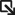• Solutions Solutions
• Products Products
• Services Services
• Support SupportSupport Portal ›
• Resources Resources
##### Networking Made Simple, Easy, and Affordable - Q&A with the Senior Director of Extreme Capital Solutions
All stories ›
• Partners Partners

# Using Operators

Operators allow you to manipulate variables and evaluate strings.

Some operators can be used in all numeric expressions, while others are restricted to integer or string expressions. The following table lists the operators supported and provides comments on when they can be used. The valid operators are listed in decreasing order of precedence.Operators

- Unary minus None of these operands can be applied to string operands, and the bit-wise NOT operand can be applied only to integers.

+

Unary plus
~ Bit-wise NOT
! Logical NOT
* Multiply None of these operands can be applied to string operands, and the remainder operand can be applied only to integers. The remainder always has the same sign as the divisor and an absolute value smaller than the divisor.
/ Divide
% Remainder
+ Add These operands are valid for any numeric operations.
- Subtract
<< Left shift These operands are valid for integer operands only. A right shift always propagates the sign bit.
>> Right shift
< Boolean less Each operator produces 1 if the condition is true, 0 otherwise. These operators can be applied to strings as well as numeric operands, in which case string comparison is used.
> Boolean greater
<= Boolean less than or equal
>= Boolean greater than or equal
== Boolean equal Each operator produces a zero or one result. These operators are valid for all operand types.
!= Boolean not equal
& Bit-wise AND This operator is valid for integer operands only.
^ Bit-wise exclusive OR This operator is valid for integer operands only.
| Bit-wise OR This operator is valid for integer operands only.
&& Logical AND This operator produces a result of 1 if both operands are non-zero. Otherwise, it produces a result of 0. This operator is valid for numeric operands only (integers or floating-point).
|| Logical OR This operator produces a result of 0 if both operands are zero. Otherwise, it produces a result of 1.This operator is valid for numeric operands only (integers or floating-point).
x?y:z If-then-else (as in the C programming language) If x evaluates to non-zero, then the result is the value of y. Otherwise the result is the value of z. The x operand must have a numeric value.